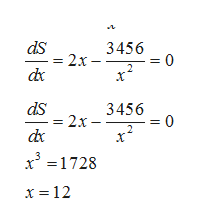# a rectangular tank that is 864 ft cubed with a square base and open top is to be constructed of sheet steel of a given thickness. find the dimensions of the tank with minimum weight

Question
29 views

a rectangular tank that is 864 ft cubed with a square base and open top is to be constructed of sheet steel of a given thickness. find the dimensions of the tank with minimum weight

check_circle

Step 1

Let the measure of side of square base of tank  is x ft and height of tank is y ft and volume of tank is 864 cubic ft. Then we have

Step 2

The problem is to minimize the surface area of tank. Surface area of tank is given by

Step 3

Now, we find dS/dx and so...help_outlineImage Transcriptioncloseds 2x dx 3456 = 0 ds - 2x dc 3456 = 0 x31728 x 12 fullscreen

### Want to see the full answer?

See Solution

#### Want to see this answer and more?

Solutions are written by subject experts who are available 24/7. Questions are typically answered within 1 hour.*

See Solution
*Response times may vary by subject and question.
Tagged in

### Derivative